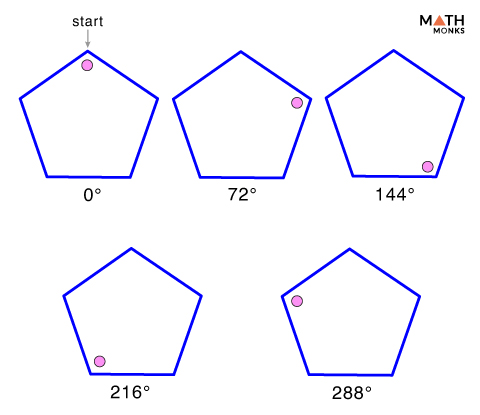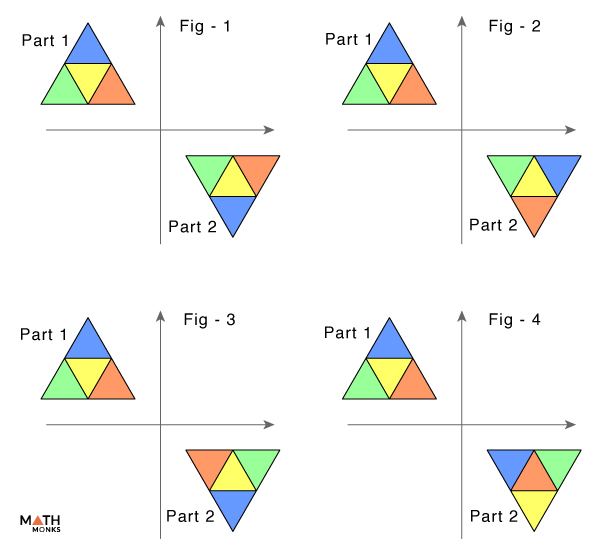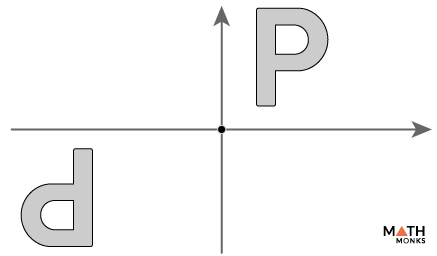# Point Symmetry

Point symmetry in a shape occurs when there is a central point on an object such that:

1. The central point divides the shape into two parts or sides, Part 1 and Part 2
1. Every point on Part 1 must have a matching point on Part 2 equidistant from the central point
2. Part 1 and Part 2 must be in opposite directions

Point Symmetry is also called Origin Symmetrysince the ‘Origin’ is the central point about which the shape is symmetrical.

Thus, when we rotate a figure about 180° and it regains its originals shape, only then we say that point symmetry exists in the figure.

## Solved ExamplesDoes a regular pentagon has point symmetry?

Solution:

Thus, a regular pentagon has one point of symmetry.Which of the given figures has a point symmetry?

Solution:

Fig -3 has point symmetry since all the parts in part 1 and part 2 figure are in opposite position with respect to each other ans also they are at equal distance from the central point.State if the given alphabet has a point of symmetry.

Solution:

No. The given alphabet does not have a point of symmetry as the two ‘P’s are not at equal distance from the central point.